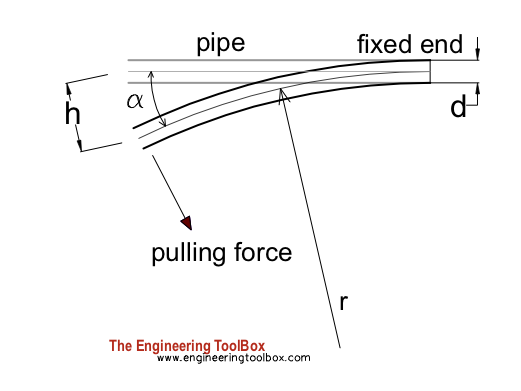Engineering ToolBox - Resources, Tools and Basic Information for Engineering and Design of Technical Applications!

Bending PE Pipes

Bending PE pipe calculator.A PE pipe is flexible and can be bended. For pressurized pipe the bending radius should not be less than

rmin = 60 d              (1)

where

rmin = minimum bending radius  (m, in)

d = pipe diameter (m, in)

For pipe without pressure - or for pressure pipes during installation - the bending radius should not be less than

rmin = 30 d           (2)

Example - Min. Radius when bending PE Pipe

The minimum bending radius for 300 mm (0.3 m) PE pipe during installation can be estimated to

rmin = 30 (0.3 m)

= 9

Radius and Angle vs. Deflection when bending Pipe

The radius of the bending pipe can be hard to measure in field. In practice a rope or chain may be tied to the end of the pipe and the end is pulled until deflection is achieved.

Deflection for a given radius and length of deflecting pipe can be calculated as

h = l2 / (2 r)                       (3)

where

h = deflection of pipe (m, in)

r = radius of bending pipe (m, in)

l = length of pipe from fixed position (m, in)

Eq. 3 can be modified with eq. 2 to calculate maximum deflection for minimum radius to

hmax = l2 / (60 d)                     (3b)

where

hmax = deflection at minimum radius (m)

The angle can be calculated as

α = l 180 / (π r)                  (4)

where

α = angle (degrees)

Eq. 4 can be modified with eq. 2 to calculate the maximum angle at minimum radius as

αmax = l 60 / (π d)                  (4b)

Bending PE Pipe Calculator

This calculator can be used to calculate maximum deflection and angle of deflection for a PE pipe during installation. It is based on eq. 3b and 4b.

d - diameter of pipe (mm)

l -  length of pipe from fixed position (m)

Related Topics

• Dimensions - Sizes and dimensions of pipes and tubes, and their fittings - inside and outside diameter, weight and more

Engineering ToolBox - SketchUp Extension - Online 3D modeling!

Add standard and customized parametric components - like flange beams, lumbers, piping, stairs and more - to your Sketchup model with the Engineering ToolBox - SketchUp Extension - enabled for use with the amazing, fun and free SketchUp Make and SketchUp Pro .Add the Engineering ToolBox extension to your SketchUp from the SketchUp Pro Sketchup Extension Warehouse!

Translate

Privacy

We don't collect information from our users. Only emails and answers are saved in our archive. Cookies are only used in the browser to improve user experience.

Some of our calculators and applications let you save application data to your local computer. These applications will - due to browser restrictions - send data between your browser and our server. We don't save this data.

Citation

• Engineering ToolBox, (2018). Bending PE Pipes. [online] Available at: https://www.engineeringtoolbox.com/pe-pipes-bending-d_2146.html [Accessed Day Mo. Year].

Modify access date.

. .

Scientific Online Calculator1 14